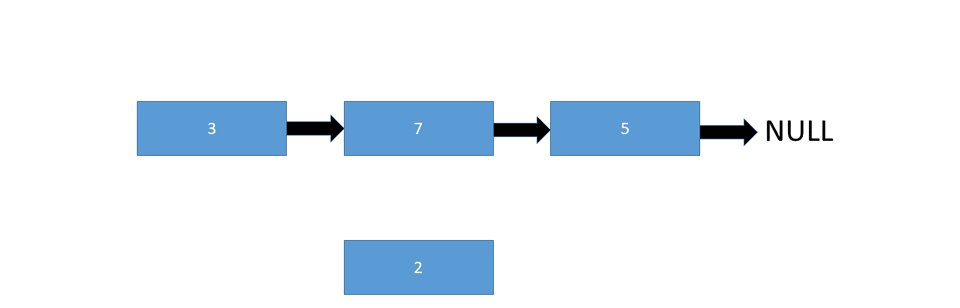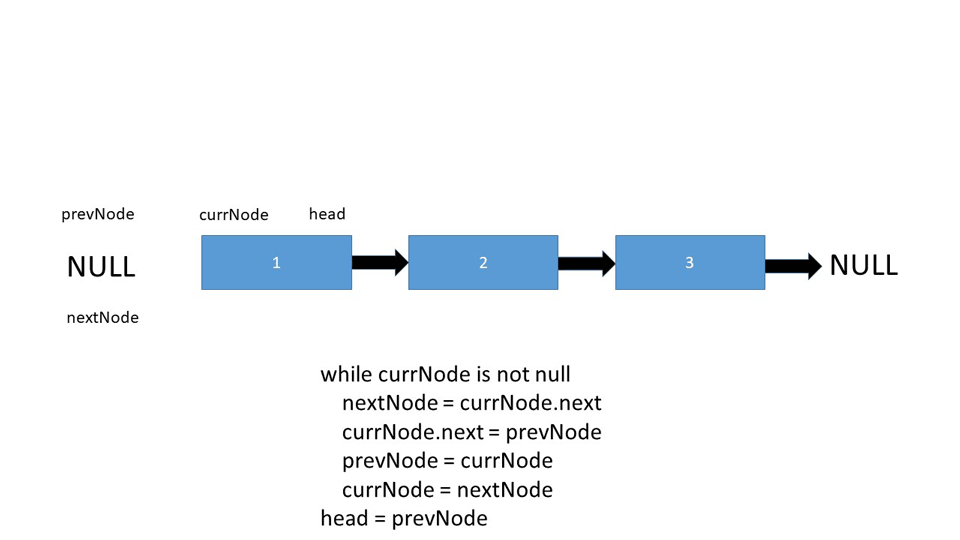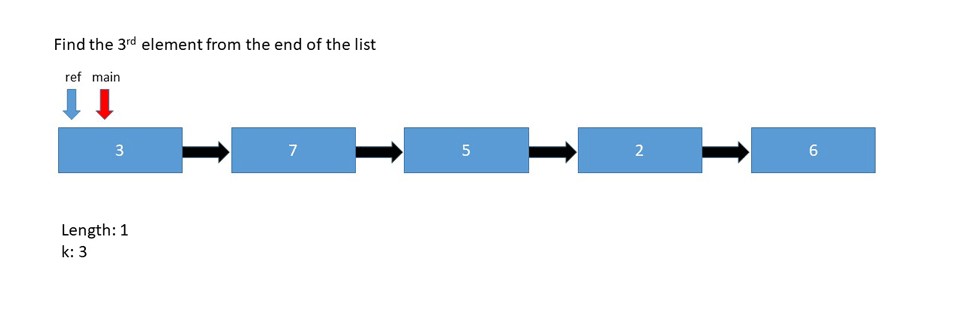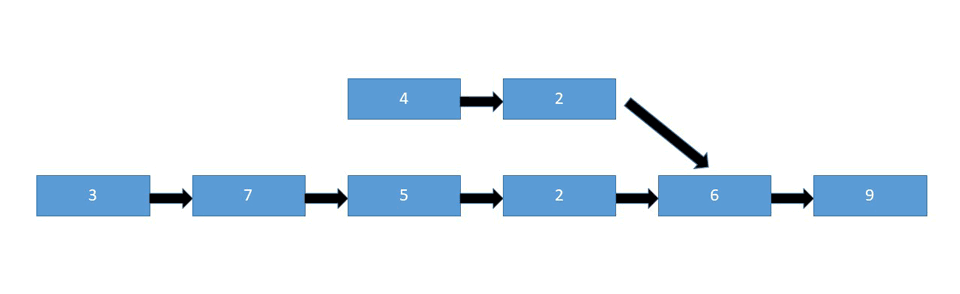Linked List Programming Interview Questions - Stack Abuse

# Linked List Programming Interview QuestionsIf you're interested in reading more about Programming Interview Questions in general, we've compiled a lengthy list of these questions, including their explanations, implementations, visual representations and applications.

### Introduction

Linked Lists are a data structure that represents a linear collection of nodes. A characteristic specific to linked lists is that the order of the nodes isn't dictated by their presence in memory, but rather the pointers that each node has to the next node in the sequence.Multiple types of Linked Lists exist:

• Singly Linked Lists - A classic linked list, like the one shown in the picture above. The nodes in Singly Linked Lists contain a pointer to the next node in the list.
• Doubly Linked Lists - This kind of Linked List contains both a pointer to the next, but also a pointer to the previous node in the list.
• Circular Linked Lists - Instead of containing a null pointer at the end of the list, the last node in these lists contains a pointer to the first node, making them circular.

As the linked list is one of the most basic, and important, data structures in computer science, we've compiled a list of common interview questions regarding linked lists for this article. Need help studying for your interview? We recommend trying a service like Daily Coding Problem, which will email you practice questions daily.

### Inserting and Removing Nodes

#### Interview Question

Remove the 4th element from the following list:

2->4->5->3->7->0


Insert an element (6) on the 2nd position in the following list:

2->4->5->3->7->0


#### Explanation

Let's start off with a simple task - inserting a node into a list. There are a few ways someone can ask you to do this:

• Inserting at the start of the linked list
• Inserting at the end of the linked list
• Inserting at a given position

Let's cover them one by one first, before diving into the process of removing a node.

#### Inserting Nodes

To insert a node at the start of a linked list, we simply need to change the current head of the linked list to the new node we're inserting, and also point the inserted node to the old head:Let's take a look at the pseudo code:

Node newNode



To insert a node at the end of a linked list, we simply need to change the last pointer from null to the new node:Let's take a look at the pseudo code:

Node newNode

while(last.next)
last = last.next
last.next = newNode1


We do this by assigning the newNode as the head, if the list is empty, and iterating to the end of the list if it isn't, adding our node.

And finally, to insert a node at the given position, we need to set the next node of the given position as the next node of the new node, and assign the new node as the next node of the given position:Let's take a look at the pseudo code:

insertAtPosition(list, node, newNode)

if node is not null
newNode.next = node.next
node.next = newNode;


#### Removing Nodes

What we have to do here is traverse the list, keeping track of the node previous from the node we want to remove.

Upon reaching the node we want to remove, we point the previous node to the node after the target node and remove the target node:

removeNode(Node node)
prevNode = null

while curr is not null
if prevNode is null
if curr == node
prevNode.next = curr.next
curr = null

prevNode = curr
curr = curr.next


### Comparing Strings Represented as Linked Lists

#### Interview Question

Write a function that can compare two Strings, represented as Linked Lists such as:

List 1: S->T->A->C->K->A->B->U->S->E
List 2: S->T->A->C->K->O->V->E->R->F->L->O->W


The function can return three values:

• 0 - If the two lists represent the same String
• 1 - If the first list is lexicographically greater than the second list
• -1 - If the second list is lexicographically greater than the first list

Note: "Lexicographically greater" means that a String would appear after the other String based on the dictionary position.

If implemented correctly, using that function on this particular example should produce the following result:

-1


#### Explanation

compare(node1, node2):

while node and node are not null AND node1.content is equal to node2.content
node1 = node1.next
node2 = node2.next

if node1 and node2 are not null
if node1.content > node2.content
return 1
else
return -1

if node1 is not null and node2 is null
return 1

if node2 is not null and node1 is null
return -1

return 0

list1 = Node('s')
list1.next = Node('t')
list1.next.next = Node('a')
list1.next.next.next = Node('c')
list1.next.next.next.next = Node('k')
list1.next.next.next.next.next = Node('a')
list1.next.next.next.next.next.next = Node('b')
list1.next.next.next.next.next.next.next = Node('u')
list1.next.next.next.next.next.next.next.next = Node('s')
list1.next.next.next.next.next.next.next.next.next = Node('e')

list2 = Node('s')
list2.next = Node('t')
list2.next.next = Node('a')
list2.next.next.next = Node('c')
list2.next.next.next.next = Node('k')
list2.next.next.next.next.next = Node('o')
list2.next.next.next.next.next.next = Node('v')
list2.next.next.next.next.next.next.next = Node('e')
list2.next.next.next.next.next.next.next.next = Node('r')
list2.next.next.next.next.next.next.next.next.next = Node('f')
list2.next.next.next.next.next.next.next.next.next.next = Node('l')
list2.next.next.next.next.next.next.next.next.next.next.next = Node('o')
list2.next.next.next.next.next.next.next.next.next.next.next.next = Node('w')

compare(list1, list2)


The while loop traverses both lists with two conditions attached:

• node1 and node2 are not null
• node1.content is equal to node2.content

This means that it will traverse both of the lists, node by node, as long as they don't reach the end of the list, and each character matches between them. If any of these two conditions return false, the loop breaks.

The if statement check whether both of the nodes aren't null:

• If the content of the first node is greater than that of the second node, we return 1
• If the content of the second node is greater than that of the first node, we return -1

And the final two if statements simply returns 1 and -1 accordingly if the list sizes don't match.

Ultimately, if it passes all the checks, it means that the lists match and represent the same String, returning 0.

### Reversing a List

#### Interview Question

1->2->3->4->5


#### Explanation

There are two ways to approach this problem: iterative and recursive.

Let's take a look at the iterative pseudo-code behind the implementation:

Node prevNode, currNode, nextNode

prevNode = null
nextNode = null

while currNode is not null
nextNode = currNode.next
currNode.next = prevNode
prevNode = currNode
currNode = nextNodeTo accomplish this, we instantiate three nodes - prevNode, nextNode and currNode. Both prevNode and nextNode are pointed to null, and currNode is set to be the current head of the linked list.

A while loop is begun with a termination condition of the currNode pointing to null, and the switching process begins:

• nextNode becomes the next node in line for the currNode

• currNode.next (the next node in line) points to prevNode, which is currently null

• prevNode is changed and now points to currNode, switching their places

• currNode is changed and now points to nextNode which was previously set to the next node in line for the currNode. This effectively advances the currNode marker through the Linked List and begins the process all over again.

• As the currNode marker moves, it eventually reaches the end and all nodes have been reversed. The termination condition is met as currNode points to null and the head becomes prevNode which is the former last node in the Linked List. Since all of the references are reversed, to complete the reversal, the head must also point to the now first element in the sequence.

With that out of the way, let's take a look at the recursive pseudo-code behind the implementation:

Node first, rest

Node recursiveReverse(Node current, previous)

if current.next is null
current.previous = previous
return
first = current.next
current.next = previous

recursiveReverse(first, current)


## Free eBook: Git Essentials

Check out our hands-on, practical guide to learning Git, with best-practices, industry-accepted standards, and included cheat sheet. Stop Googling Git commands and actually learn it!

Return a random node from a given linked list ensuring that the probability to fetch that specific node is 1/n where n is the size of the list:

1->4->2->5->9->7


#### Explanation

There are two ways to approach this problem and come up with a solution:

• Traversing the list and counting as we go. Then we traverse it again, selecting each and every node with probability 1/n before we generate a random number from 0 to N-i for the i'th node and selecting the node if the generated number is equal to 0 or any other fixed number from the 0 to N-i range.

This approach has a major setback - we need to traverse the list twice. Once to count it and another time to select a random node. In most cases, this approach isn't wanted, so we turn to the other one:

• Reservoir Sampling - We select the first element, regardless of the list size since we don't already know it in advance. Then, we traverse the list forward considering each and every subsequent node for selection with the probability 1/n where n is the number of already visited nodes. This way, we ensure that the probability of choosing any given node from the list is 1/n.

Note: This is in fact a simplified version of Reservoir Sampling, which is often used for selecting k elements from a list containing n items where n is unknown or very large. In this case, we're also selecting k elements, which happens to be a single element.

Let's take a look at the pseudo-code behind the implementation:

reservoirSample(stream, n, k)
i = 0
// initialize reservoir with k elements
reservoir[k]

// populate the reservoir with the wanted number of elements
for i < k
reservoir[i] = stream[i]

// iterate from the (k+1)th element to nth element
while i < n
// pick random number from 0 to i
j = randomNumber(i+1)
// if the random index is smaller than k, replace the element at that index with a new element from te stream
if j < k
reservoir[j] = stream[i]


### Finding the Middle Node

#### Interview Question

##### Even Number of Elements

Find the middle node in the given linked list:

1->2->3->4->5->6

##### Uneven Number of Elements

Find the middle node in the given linked list:

1->2->3->4->5


#### Explanation

This question can have two versions:

• The linked list has an even number of elements - We're looking for the two middle elements since there's no definitive middle element, after which we'll select the second middle element
• The linked list has an uneven number of elements - We're looking for the middle element

Additionally, we also have two approaches:

• Count the nodes in the list, divide the number by 2 and return the node at that position.
• Traverse the list with two pointers - the first one increments by 1, while the second one increments by 2. This way, the second one will reach the end of the list when the first one is in the middle.

Let's start off with the pseudo code behind the first approach:

int count

count+1
return count

list.getNode(count/2)


With that out of the way, let's explore the pseudo code behind the second approach:

Node slow, fast

while(fast is not null && fast.next is not null)
fast = fast.next.next
slow = slow.next
return slow### Kth Element from Last Node

#### Interview Question

Find the 5th element from the end of the linked list:

1->2->4->6->7->8->5->9


#### Explanation

In the case above, we're looking for the node with the value 6, since it's the 5th node looking back from the end of the list.

There are two ways to get to this node:

• Relying on the length of the list - (Length - n + 1)th node from the beginning.
• Using pointers - A reference pointer and a main pointer. Both pointers will start at the head of the list, with the reference pointer shifted by K nodes along the list. Then both pointers iterate by 1 until the reference pointer reaches the end. This makes the main pointer fall behind the reference pointer by K node, pointing at the node we're looking for.

Let's look at the pseudo code if we're relying on the length of the list:

printKthFromLast(int k)
length = 0

// traverse the list and count the elements
while node is not null
node = node.next
length+1
// if the kth element is larger than the length, return the head
if length < k
return

// traverse the list until the element we're searching for and return it
for i = 0, i < length-k+1, i+1
node = node.next
return nodeLet's look at the pseudo code if we're relying on the pointers:

printKthFromLast(int k)

// since we're already on the head, the length is equal to 1
length = 1
while length < k
// checks if we've reached the end
if reference is null
return

reference = reference.next
length+1
while reference is not null
main = main.next
reference = reference.next
return main### Frequency of a Given Number

#### Interview Question

Count the number of times a number has occurred in a given linked list:

1->2->1->3->1->4->1->5


#### Explanation

This is both a common and a simple problem to encounter and tackle, and there's only a couple of steps needed to take in order to come up with a solution:

• Declare a counter
• Traverse each element of the list, and increase the counter every time an element is equal to the number whose frequency we're counting
• Return the count

Let's take a look at the pseudo-code behind the implementation:

count(searchFor)
count = 0

while curr is not null
if curr.data == searchFor
count++
curr = curr.next

return count


Implementing the pseudo-code in any language and running it with this list would yield:

4


### Intersection of Two Linked Lists

#### Interview Question

Given two linked lists, where a node in the first one points to a node in the second one, in a random place, find the intersection place of these two lists.#### Explanation

There are several approaches you could take here:

• Brute Force - As the name implies, in a brute force fashion, traverse the entire second list, for each node in the first list and check for the intersection. This approach takes the most time, so it's very inefficient to use with large lists.
• Hash Table - Traverse the first list and store an address for each node in a hash set. Then traverse the second list and if an address from the second list is already stored in the hash set, it's the intersection node.
• Two Pointers - Again, using two pointers, we can find the intersection node. Initialize two pointers, pointerA and pointerB where both pointers traverse the list one step at a time. One list must be smaller than the other. If they had the same number of nodes and connected at the end, it'd simply be a singly linked list. If they have the same number of nodes, the list that connects to the other list before the end becomes the "bigger" list since it has more individual nodes. When pointerA reaches the end of the list, redirect it to the head of the second list. If at any point these two pointers meet, that's the intersection node. If it's a bit confusing, it might be better to take a look at the attached animation below:Let's first take a look at the pseudo-code behind the brute force approach:

Node list1_curr = head1

while list1_curr is not null
while list2_curr is not null
if list1_curr == list2_curr
return list1_curr


Now, let's take a look at the pseudo-code behind the hash table approach:

getIntersectionHashTables(Node head1, Node head2)
nodes = hashSet

while pointerA is not null
pointerA = pointerA.next

if nodes is empty
return null

while pointerB is not null
if nodes contain pointerB
return pointerB
pointerB = pointerB.next
return null


This one is quite simple as well:

• We add all the nodes from the first list into a hash set and then traverse the second list, checking if the hash table contains such a node already.
• If the hash table contains the node, it's returned and if not, null is returned.

Finally, let's take a look at the two pointers approach:

if pointerA is null and pointerB is null
return null

while pointerA is not pointerB
pointerA = pointerA.next
pointerB = pointerB.next

if pointerA is null
if pointerB is null

return pointerA


### Resources

If you're looking for a better way to practice these kinds of programming interview questions, as well as others, then we'd recommend trying out a service like Daily Coding Problem. They'll send you a new problem to solve every day, all of which come from top companies. You can also find out more here if you want more info.

### Conclusion

Again, if you're interested in reading more about Programming Interview Questions in general, we've compiled a lengthy list of these questions, including their explanations, implementations, visual representations and applications.

Last Updated: September 17th, 2019

Get tutorials, guides, and dev jobs in your inbox.

## Prepping for an interview?

• Improve your skills by solving one coding problem every day
• Get the solutions the next morning via email
• Practice on actual problems asked by top companies, like:

## Better understand your data with visualizations

•  30-day no-questions refunds